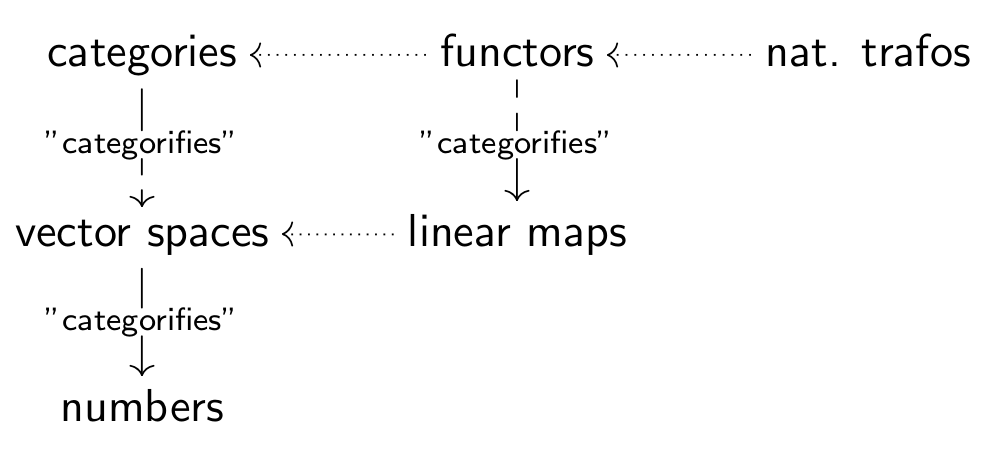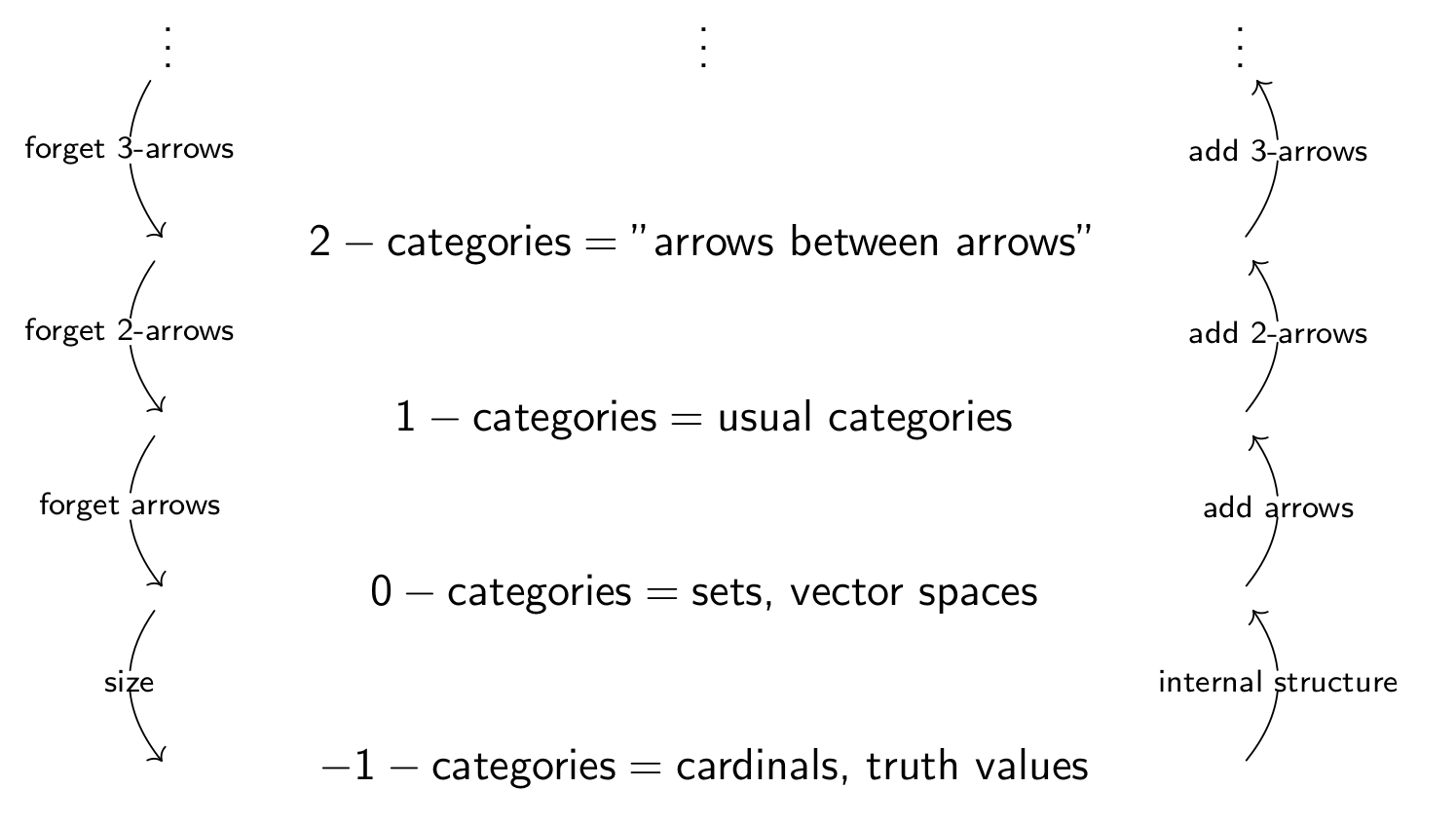## One diagram is worth a thousand words

Each step of a “categorification process” should reveal more structure. The most classical illustration of this is the following:Here we first “categorify” numbers into vector spaces. The new information available are now linear maps between vector spaces (thus, we have the whole power of linear algebra at hand). There is no reason to stop: we can “categorify” vector spaces into categories, linear maps into functors. Again, we see a new layer of information, namely the natural transformations between these functors. The last step “naturally lives” in a 2-categorical setup. The idea is clear: keep on going (if possible of course).
Let me give now some more details.

## A rough description

Since categorification is a rather new subject of mathematics (well, it is not the “newest kid in the block”, but anyway), I should spend a few words about the motivation and ideas behind it. For more information, one can look at the nice introduction at n-lab link.
The idea behind categorification is, given a fixed notion one really likes, to find an “explanation” for properties of this structure by considering natural construction in a category such that the structure is some kind of shadow of these constructions. To this end, one replaces “set like structures” (i.e. 0-categories) with “category like structures” (i.e. 1-categories). Of course one can perform such a process on any level, e.g. one can categorify a “n-category like structure” into a “(n+1)-category like structure”. Or one can “categorify with extra structure”, e.g. categorify vector spaces instead of sets.
Or, to say it otherwise, categorification is an “inverse” process for decategorification (which is best to be defined via examples)
Some examples of decategorification are (note that the notion categorification is too new and therefore not directly used in these classical examples):

• The category of finite sets is a categorification of the natural numbers. Decategorification is just counting.
• But categorifications are not unique, i.e. the category of finite-dimensional vector spaces can also be seen as a categorification of the natural numbers. Decategorification is taking dimensions.
• The homology groups of a reasonable space are a categorification of its Betti numbers (Noether, Hopf, Walther). Decategorification is taking dimensions.
• The Khovanov homology is a categorification of the Jones polynomial. Decategorification is taking q-graded dimensions.
• A topos (Lawvere) can be seen as a categorification of a Heyting algebra.